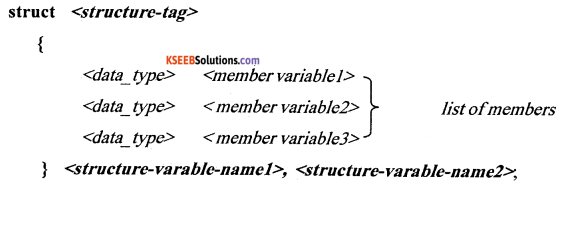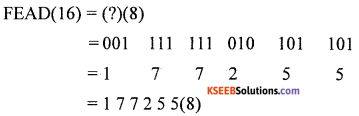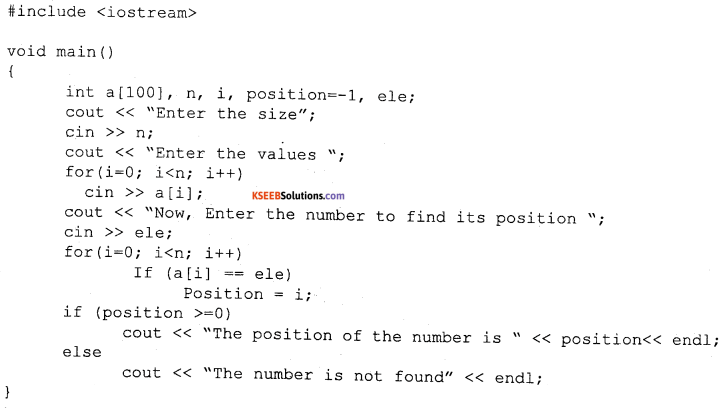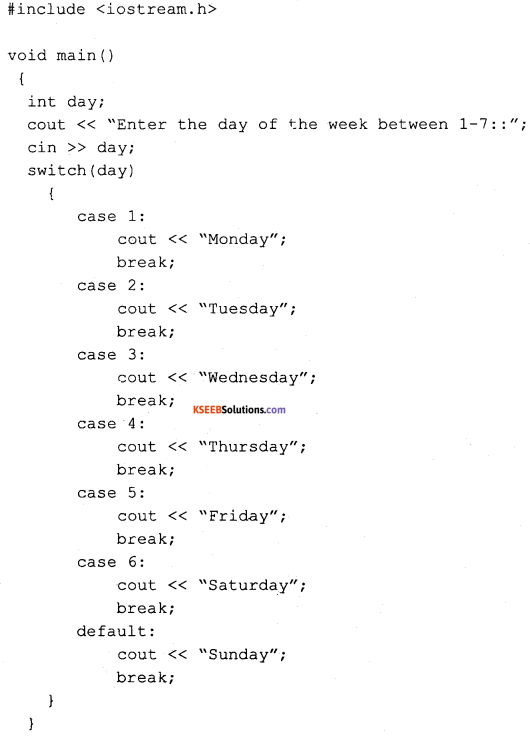# 1st PUC Computer Science Previous Year Question Paper March 2014 (North)

Students can Download 1st PUC Computer Science Previous Year Question Paper March 2014 (North), Karnataka 1st PUC Computer Science Model Question Papers with Answers helps you to revise the complete Karnataka State Board Syllabus and score more marks in your examinations.

## Karnataka 1st PUC Computer Science Previous Year Question Paper March 2014 (North)

Time: 3:15 Hours
Max. Marks: 100

PART – A

I. Answer all the following questions. Each question carries one mark. (10 × 1 = 10)

Question 1.
Which is the earliest computing machine?
The earliest computing machine was Abacus.

Question 2.
Give anyone example of non-impact printer?
One example of non-impact printer is Ink jet printer.

Question 3.
What is syntax error?
It refers to an error in the syntax of a sequence of characters or tokens that is intended to be written in a particular programming language.

Question 4.
Define keyword.
All keywords (reserved words) are basically the sequences of characters that have one or more fixed meanings.Question 5.
What is the extraction operator in C++?
cin is used in conjuction with >> operator, known as extraction or get from operator in C++.

Question 6.
What is the purpose of break statement?
The break statement can be used to terminate a repeated structure (loops) such as while, do while and for and multi branching statements like switch.

Question 7.
Name the data type of the array subscript.
The data type of array subscript is integer or int.

Question 8.
Define actual arguments.
The data that is passed by the calling function as arguments/parameters is known as actual arguments, i.e., the arguments which are present at the time of function call.

Question 9.
Name any two word processors.
The two word processors are MS word, Word perfect.Question 10.
What is a worksheet?
It is a sheet that contains rows and columns, to store data like student marks, salary of employees, etc.,.

PART – B

II. Answer any Five of the following questions. Each question carries two marks: (5 × 2 = 10)

Question 11.
How are computers important in communication?
The World Wide Web, Internet, Social Networking and email are very useful in communication. They work very fast and available 24/7.

Question 12.
Compare static RAM and dynamic RAM.

1. In a Static RAM, each bit that stores data is made up of four or six transistors that make up a flip-flop.
2. In a dynamic RAM, uses separate capacitors to store each bit. Capacitors when not charged represent the value 0 of a bit and when charged represent the value 1.

Question 13.
Define the terms Multiprogramming and multitasking.
In multiprogramming, several programs are run at the same time on one processor. The operating system executes part of one program, then part of another, and so on.

Running two or more programs at the same time on the same single-processor (single CPU) computer is called multitasking. Multitasking is the logical extension of multiprogramming.

Question 14.
Mention any two advantages of the flowchart.

1. It is a means of communication and easy to understand.
2. Easy to convert into a program code.Question 15.
Write any two characteristics of OOPs.
Data encapsulation and inheritance are the two important characteristics of OOP.

Question 16.
What are variables? Give its declaration syntax.

1. It is a location in the computer memory which can store data and is given a symbolic name for easy reference.
2. The declaration syntax is datatype variablename;

Question 17.
Explain any two character based functions.
tolower(): It converts the capital alphabet character to lower case.
For example, tolower(‘A’) output: a

toupper ( ): It converts the lower case alphabet character to upper case.
For example, toupper(‘a’) output: AQuestion 18.
What are headers in footers in word processor?
A header is a special area at the top of every page normally allocated in the top margin area. Footer is a special area at the bottom of every page normally allocated in the bottom margin area.

PART – C

III. Answer any Five of the following Questions. Each question carries three marks: (5 × 3 = 15)

Question 19.
Give the application of OCR, OMR, MICR devices.
Optical Character Recognition (OCR) is a input device that is used to scan the printed/handwritten text materials into computer readable text makes it easily edited and stored.

Optical Mark Recognition (OMR) is the technology of electronically taking out data from marked fields, such as oval shades and fill-in fields, on printed forms.

Magnetic Ink Character Reader enables special characters printed in magnetic ink to be read and input rapidly to a computer.

Question 20.
Explain the features of UNIX operating system.
The features of UNIX operating system are
more than one program can be run at a time.

2. Machine-independence:
The System hides the machine architecture from the user, making it easier to write applications that can run on micros, mini and mainframe computers.

3. Multi-User Operations:
UNIX is a multi-user system designed to support a group of users simultaneously. The system allows for the sharing of processing power and peripheral resources, white at the same time providing excellent security features.Question 21.
Define

1. Testing
2. Debugging
3. Top-down analysis.

1. Testing:
It is the process of checking the program logic by providing selected sample data and observing the output for correctness.

2. Debugging:
The process of detecting the errors and correcting the errors in a program is called as debugging.

3. Top down analysis:
It is the process of dividing a problem into sub problems and further dividing the sub problems into smaller sub problems and finally implemented as program statements.

Question 22.
Write an algorithm to find largest of three numbers.

• Step 1: start
• Step 2: input a, b, c
• Step 3: let max = a
• Step 4: is b > max? then max = b
• Step 5: is c > max? then max = c
• Step 6: print max
• Step 7: stop

Question 23.
Explain the different relational operators with examples.
The operators which perform operation of relation between two operands called relational operators.
The > (greater than) operator: if (10 > 5)? Output: True. If ( 5 > 10)? Output: False
The < (lesser than) operator: if (15 < 25)? Output: True. If(56<26)? Output: False
The >= (greater than or equal to): if (10 >= 10)? Output: True. If ( 5 >= 10)? Output: FalseQuestion 24.
What is cascading of input and output operators? Explain with suitable examples.
The cascading is a way to extract/insert multiple values from/into more than one variable using one cin/cout statement. The multiple use of << or >> in one statement is known of cascading.

1. Cascading of output operator (>>):
cout << ” Hello ” << ” ISC “;
cout << “Value of B=” << b;

2. Cascading of input operator (>>):
int n1, n2, n3;
cin >> n1 » n2 >> n3;
cin >> n1 >> n2;

Question 25.
Write the syntax and examples of initialization of two-dimensional array?
Syntax of initialization of two dimensional array:
Datatype arrayname [rowsize] [column size] = { value 1, value2, …., value n };
Example of array initialization with declaration:
int matrix   = { 91, 96, 90, 94, 99, 95, 87, 92, 98};

Question 26.
How is structure defined? Write syntax and example.
A structure is a collection of variable which can be same or different types.Example,
struct student
{
int, regno;
char name;
char class [ 6];
};

PART – D

IV. Answer any Seven of the following questions. Each question carries Five marks: (7 × 5 = 35)

Question 27.
Explain the characteristics of computers in detail.
The characteristics of computers are
1. Speed:
Computers are fast in doing calculations. The speed of the computer is measured in terms of million instruction per second (MIPS).

2. Storage Capacity:
Computers come with large amount of memory. They can hold lot of data. Computers can show a particular piece of information from large amount of data in a short time.

3. Diligence:
After doing work for sometime, humans become tired but computers do not become tired. They work continuously. In fact, some computers which control telephone exchanges are never stopped. This is called diligence.

4. Accuracy:
The results that the computers produce are accurate provided data and programs are reliable.

5. Versatility:
We can use computer to perform completely different type of work at the same time.

6. Reliability:
Computers work for many years without any problem. Few computers in the world are never switched off.Question 28.
Solve: FEAD(16) = (?)(10) = (?)(2) = (?) (8)
FEAD(16)= (?)(10)= 15 × 163 + 14 × 162 + 10 × 161 + 13 × 160
= 61440 + 3584+ 160 + 13
= 65197(10)
In hexdecimal system F=15, E=14, A=10 and D= 33
15 = 1111(2) 14 = 1110(2) 10 = 1010(2) 13 = 1101(2)
FEAD(16) = (?)(2)= 1111 1110 1010 1101(2)>

Question 29.
What is structured programming? Mention its advantages.
Structured Programming deals only with logic and code and suggests making use of programming structures such as sequence, selection, iteration and modularity in programs.

1. Programs are easy to write because the programming logic is well organized.
2. Programs can be functionally decomposed into logical working units (modularity).
3. Modularity leads to easily understand the program, test and debug.
4. Easy to maintain because of single entry and single exit.
5. Eliminates the use of undisciplined controls (GOTO, BREAK, etc.,) in the program.

Question 30.
Draw a flowchart to find the factorial of a number.Question 31.
Explain the structure of C++program with an example.The various parts of the above program:
1. headers, which contain information that is either necessary or useful to program. For this program, the header <iostream> is needed.

2. The line int main() is the main function where program execution begins.

3. The pair of { } indicates the body of the main function.

4. The next line cout << “This is my first C++ program. causes the message “This is my first C++ program” to be displayed on the screen.

5. The next line return 0; terminates main( )function and causes it to return the value 0 to the calling process.Question 32.
Write a C++ program to find the position of a given number in an array.Question 33.
Explain the working of switch statement with an example.
Switch statement compares the value of an expression against a list of integers or character constants. The list of constants are listed using the “case” statement along with a “break” statement to end the execution.Result:
Enter the day of the week between 1 – 7 :: 7
Sunday

In the above Control Structure example the “switch” statement is used to find the day of the week from the integer input got from the user. The value present in the day is compared for equality with constants written in the word case. Since no equality is achieved in the above example (from 1 to 6) as they entered value is 7, default is selected and gives “Sunday” as a result.Question 34.
Explain the working of for statement with a programming example.
A for loop is a repetition control structure that allows you to efficiently write a loop that needs , to execute a specific number of times.
Syntax:
The syntax of a for loop in C++ is:
for ( initialization; condition; increment/decrement )
{
statement(s);
}

The working of a for loop:
1. The initialization step is executed first, and only once in the beginning. It is used to declare and initialize any loop control variables.

2. Next, the condition is evaluated. If it is true, the body of the loop is executed. If it is false, the body of the loop does not execute and flow of control jumps to the next statement just after the for loop.

3. After the body of the for loop executes, the flow of control jumps back up to the increment/decrement statement and update any loop control variables.

4. Then condition is evaluated again. If it is true, the loop executes and the process repeats itself (body of loop, then increment step, and then again condition). After the condition becomes false, the for loop terminates.

Example:<

When the above code is compiled and executed, it produces following result:
value of a: 10
value of a: 11
value of a: 12
value of a: 13
value of a: 14
value of a: 15

Question 35.
What is recursive function? Explain with a programming example.
A function that calls itself directly or indirectly again and again is called recursive functions and the process is termed as recursion. The most common example of a recursive function is the calculation of the factorial of a number. i.e., n! = (n) * (n – 1)
Program:In the above example, the calling function main () gives the function call to fact () and control jumps from main () function to called function fact().

The argument ‘n’ value is compared with the base class ‘if (n == 1)’ if ‘true’ control will return back to calling function main() with the value 1.

If ‘False’, control will execute the statement which is after ‘else’ x = n * fact ( n – 1);. here a function call is given to fact (n-1) with the parameter (n-1).

Now the calling function is fact () and called function is also fact(). The recursion ends when value of ‘n’ is 1 then the statement return (1) is initiated.Question 36.
Spreadsheets are mainly used for making calculations and mathematical works. Microsoft excel is a spread sheet application. In a single sheet, it consists of rows and columns and cells. Every cell has different address.

1. Tables:
Tables are created with different fields eg – name, age, address, roll no so we add a table to fill these values;

2. Clip art:
In this we can add images and also audio, video clips can be added here.

3. Charts:
With charts, we can clearly shown products evaluation to the clients. For example which product sale is more or less in this month.

4. Functions:
LOGICAL: average, sum, mod, product.

5. Images and Backgrounds:
In this we add images and backgrounds in sheet.

6. Sorting and Filter:
In sorting we can sort our data and also filter our data so that repetitions will be removed.

7. Page layout:
In this themes, colors, sheets, margins, size, backgrounds, breaks, print, titles, sheets height, width, scaling, gridness, headings, views, bring to front of font or back alignment, etc will be used.Question 37.
Explain the basic tags in HTML with examples (any five tags).
1. Bold Text:
You can bold text to emphasize information on your web page. Bold text is useful for introducing new terms and highlighting important phrases on a Web page.
Tag:
<B> SOME TEXT </B>
EXAMPLE:
<BODY> <B> THIS IS A Bold TEXT </B> </BODY>

2. Underline Text:
Tag:
<U> Some text </U>
Example:
<BODY> <U> You can underline this text </U> </BODY>

3. Italicise Text:
Tag:
<I> SOME TEXT </I>
EXAMPLE:
<BODY> <I> THIS IS A Itilicize TEXT </I> </BODY>

4. Big Text:
Tag:
<Big> SOME TEXT </Big>
EXAMPLE:
<BODY> <Big> THIS IS A Big TEXT</Big> </BODY>

5. Marquee Text:
To scroll text horizontally.
Tag:
<Marquee> some text </marquee>
Example:
<marquee> The text is moving < /marquee>

error: Content is protected !!